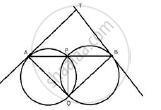Share

# Two Circles Intersect in Points P and Q. a Secant Passing Through P Intersects the Circles in Aand B Respectively. Tangents to the Circles at a and B Intersect at T. A, Q, B and T Lie on a Circle. - Mathematics

Course

#### Question

Two circles intersect in points P and Q. A secant passing through P intersects the circles in Aand B respectively. Tangents to the circles at A and B intersect at T. Prove that A, Q, B and T lie on a circle.

#### SolutionJoin PQ.
AT is tangent and AP is a chord.
∴ ∠TAP = ∠AQP(angles in alternate segments) ........(i)
Similarly, ∠TBP =  ∠BQP .......(ii)
∠TAP + ∠TBP = ∠AQP  + ∠BQP
⇒ ∠TAP + ∠TBP  =  ∠AQB ………(iii)
Now in ΔTAB,
∠ATB + ∠TAP  + ∠TBP = 180°
⇒  ∠ATB + ∠AQB = 180°
Therefore, AQBT is a cyclic quadrilateral.
Hence, A, Q, B and T lie on a circle.

Is there an error in this question or solution?